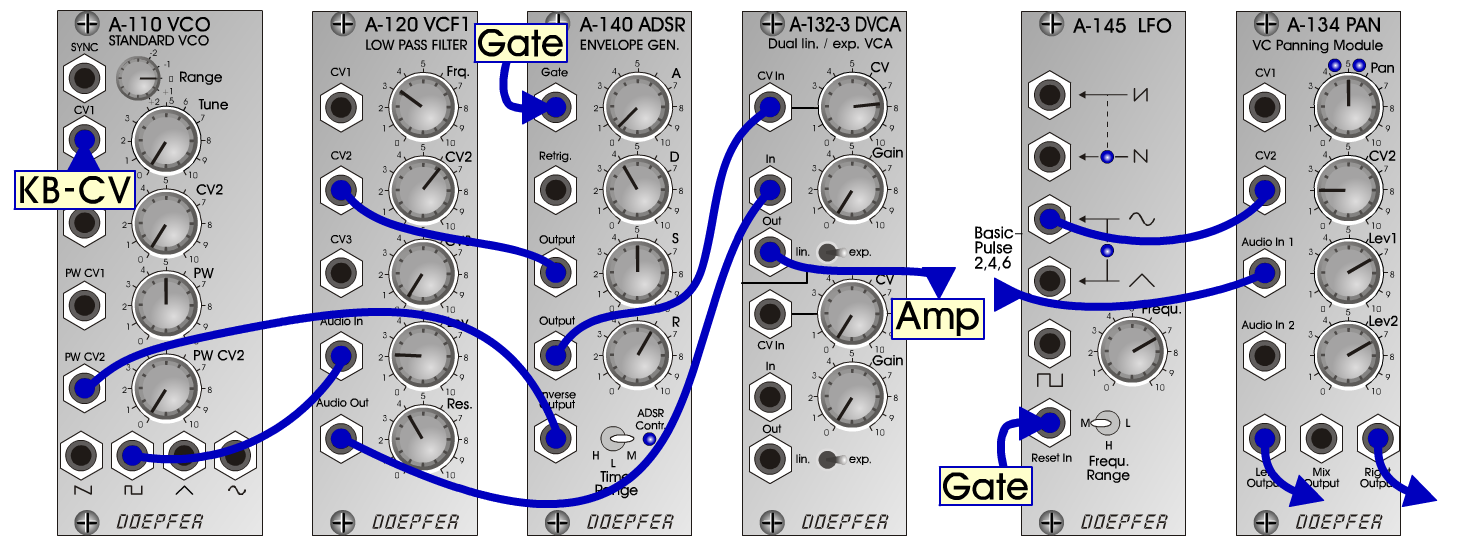Basic-PulseS y n t hD o e p f e r A - 100 Connections Basic-Pulse 1, 3, 5, 7: Settings: A-110 (Pulse) <=> A-120 (Audio In)  A-120 (Audio Out) <=> A-132-3 (In)  A-140 (Output) <=> A-132-3 (CV In)  A-140 (Output) <=> A-120 (CV2)  A-140 (Inverse Output) <=> A-110 (PW CV2)  A-132-3 (Out) <=> Amp A-110 (PW = 5, PW CV 2 = 0)  A-120 (Freq = 3.2, CV2 = 6.3, Lev = 2.1, Res = 4)  A-140 (A = 0.5, D = 4, S = 5, R = 6, Range = M)  A-132-3 (CV = 7.8, Gain = 0, exp.) Connections Basic-Pulse 2, 4, 6: Basic-Pulse2: A-110 (Pulse) <=> A-120 (Audio In)  A-120 (Audio Out) <=> A-132-3 (In)  A-140 (Output) <=> A-132-3 (CV In)  A-140 (Output) <=> A-120 (CV2)  A-140 (Inverse Output) <=> A-110 (PW CV2)  A-145 (Sine) <=> A-134 (CV2)  A-132-3 (Out) <=> A-134 (Audio In 1)  A-134 (Left Output) <=> Amp Left  A-134 (Right Output) <=> Amp Right A-110 (PW = 5, PW CV 2 = 0)  A-120 (Freq = 3.2, CV2 = 6.3, Lev = 2.1, Res = 4)  A-140 (A = 0.5, D = 4, S = 5, R = 6, Range = M)  A-132-3 (CV = 7.8, Gain = 0, exp.)  A-145 (Frq = 7, Range = M)  A-134 (Pan = 5, CV 2 = 2, Lev 1 = 7, Lev 2 = 7) Basic-Pulse3: A-110 (PW = 5, PW CV 2 = 2)  A-120 (Freq = 3.2, CV2 = 6.3, Lev = 2.1, Res = 4)  A-140 (A = 0.5, D = 4, S = 5, R = 6, Range = M)  A-132-3 (CV = 7.8, Gain = 0, exp.) Basic-Pulse4: A-110 (PW = 5, PW CV 2 = 2)  A-120 (Freq = 3.2, CV2 = 6.3, Lev = 2.1, Res = 4)  A-140 (A = 0.5, D = 4, S = 5, R = 6, Range = M)  A-132-3 (CV = 7.8, Gain = 0, exp.)  A-145 (Frq = 7, Range = M)  A-134 (Pan = 5, CV 2 = 2, Lev 1 = 7, Lev 2 = 7) Basic-Pulse5: A-110 (PW = 5, PW CV 2 = 2)  A-120 (Freq = 3.2, CV2 = 4, Lev = 2.1, Res = 4)  A-140 (A = 2, D = 4, S = 5, R = 4, Range = M)  A-132-3 (CV = 7.8, Gain = 0, exp.) Basic-Pulse6: A-110 (PW = 5, PW CV 2 = 2)  A-120 (Freq = 3.2, CV2 = 4, Lev = 2.1, Res = 4)  A-140 (A = 2, D = 4, S = 5, R = 4, Range = M)  A-132-3 (CV = 7.8, Gain = 0, exp.)  A-145 (Frq = 7, Range = M)  A-134 (Pan = 5, CV 2 = 2, Lev 1 = 7, Lev 2 = 7) Basic-Pulse7: A-110 (PW = 5, PW CV 2 = 4)  A-120 (Freq = , CV2 = 0, Lev = 2.1, Res = 7)  A-140 (A = 0.7, D = 4, S = 3, R = 3, Range = M)  A-132-3 (CV = 7, Gain = 0, exp.) Josef MuellerSound samples Bach Prelude c-moll (BWV 997)     (Basic-Pulse 2,4,6) Basic-Pulse 1 A Basic-Pulse 1 B Basic-Pulse 1 C Basic-Pulse 1 D Basic-Pulse 2 A Basic-Pulse 2 B Basic-Pulse 2 C Basic-Pulse 2 D Basic-Pulse 3 A Basic-Pulse 3 B Basic-Pulse 3 C Basic-Pulse 3 D Basic-Pulse 4 A Basic-Pulse 4 B Basic-Pulse 4 C Basic-Pulse 4 D Basic-Pulse 5 A Basic-Pulse 5 B Basic-Pulse 5 C Basic-Pulse 5 D Basic-Pulse 6 A Basic-Pulse 6 B Basic-Pulse 6 C Basic-Pulse 6 D Basic-Pulse 7 A Basic-Pulse 7 B Basic-Pulse 7 C Basic-Pulse 7 D